# Bliedtner-Hansen lemma

(Redirected from Bliedtner–Hansen lemma)

Letbe a subset of a Euclidean space,, and letbe a positive superharmonic (lower semi-continuous) function on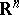(cf. also Harmonic function; Semi-continuous function). The balayageofonis defined as the greatest lower semi-continuous minorant of the function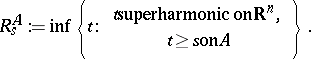Balayaged functions were introduced in classical potential theory by M. Brelot and play an important role. Given any setand any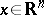, there is a unique Radon measureonsuch that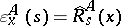for any superharmonic function. It can proved that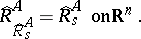(a1)

If, now,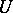is a bounded open subset of,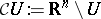, andis a Borel function on the boundary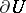of, then the function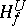: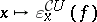, which is harmonic on, represents a generalized solution of the classical Dirichlet problem. According to (a1), "the solution of a solution is again a solution" . More precisely, given a (bounded) Borel functionon, then the function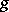: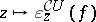,, is a Borel function andfor any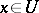.

The last assertion, as well (a1), fails to be valid in more general situations such as, for example, the case of solutions of the heat equation. Replace nowby an abstract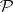-harmonic space (in the axiomatic sense of C. Constantinescu and A. Cornea; see [a4]). By this one understands a locally compact spacehaving a countable base equipped with a sheafof "harmonic" functions. Main model examples of abstract harmonic spaces are described by solutions of the Laplace equation inand of the heat equation in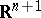. Notice also that (a1) expresses nothing else than the axiom of polarity, and this axiom is fulfilled for the first model but fails for the second one.

One may introduced ona class of superharmonic functions in a natural way and, as above, one may define balayages of superharmonic functions. As superharmonic functions are lower semi-continuous only, one defines the fine topology onas the weak topology ongenerated by the family of all superharmonic functions. This new topology was introduced in classical potential theory by Brelot and H. Cartan around 1940 and later on intensively studied, even in the axiomatic setting of harmonic spaces.

As mentioned above, (a1) is no more valid in abstract harmonic spaces without the presence of the axiom of polarity. Nevertheless, the fundamental Bliedtner–Hansen lemma, which is closely related to (a1), allows one to derive strong results in abstract harmonic spaces. It says that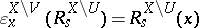, providedandare open in the fine topology,is a Borel set in the original topology ofand.

The powerful result of J. Bliedtner and W. Hansen can be stated in various degrees of generality. It appeared originally in [a1] and can be proved also in the framework of balayage spaces [a2] or even of standard-cones [a3]. Detailed proofs of the Bliedtner–Hansen lemma can be found in [a5].

How to Cite This Entry:
Bliedtner–Hansen lemma. Encyclopedia of Mathematics. URL: http://encyclopediaofmath.org/index.php?title=Bliedtner%E2%80%93Hansen_lemma&oldid=22144